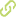The relationship between percent time <70 mg/dL and percent time <54 mg/dL measured by continuous glucose monitoring Journal ArticleOverview
abstract
• Objective: While it is recognized that there is a strong relationship between the amount of time glucose levels are <70 mg/dL (T(<70)) and the amount of time <54 mg/dL (T(<54)), the association has not been well quantified. Methods: Datasets with Dexcom continuous glucose monitoring (CGM) data from nine type 1 diabetes randomized trials were pooled to evaluate the relationship between CGM-measured T(<70) and T(<54). Penalized B-spline regression lines were fitted to assess the relationship between T(<70) and T(<54) for blinded CGM use, unblinded CGM use without an automated insulin delivery (AID) system, and unblinded CGM use with an AID system. Results: For blinded data, the T(<54) : T(<70) ratio varied from 19% when the amount of T(<70) was <1% to 44% when the amount of T(<70) was ≥7% whereas for unblinded data the ratio varied from 15% to 42%, respectively. When T(<70) was 4%, the predicted T(<54) was 1.18%, 0.94%, and 0.91% for the blinded, unblinded, and AID data, respectively (P<0.001 comparing blinded versus unblinded and AID). Conclusions: The T(<54) : T(<70) ratio increases with greater T(<70), and the ratio generally is higher with blinded than unblinded CGM data, with the latter appearing to be similar to AID system data. The finding of greater T(<54) for a given T(<70) with blinded CGM data is presumed to be due to an action being taken by the unblinded CGM user and/or by the AID system to minimize hypoglycemia which will have the effect of reducing the amount of T(<54).

authors
publication date
• 2023
• published in
Research
keywords
• Blood
• Diabetes
• Drugs and Drug Therapy
• Monitoring, Physiologic
• Randomized Controlled Trials